# Volume Mass Density Volume is the amount of

• Slides: 10Volume, Mass & Density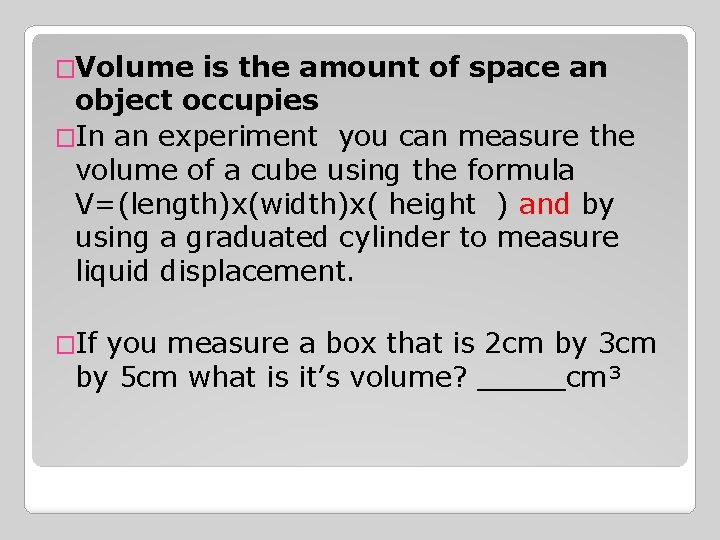�Volume is the amount of space an object occupies �In an experiment you can measure the volume of a cube using the formula V=(length)x(width)x( height ) and by using a graduated cylinder to measure liquid displacement. �If you measure a box that is 2 cm by 3 cm by 5 cm what is it’s volume? _____cm³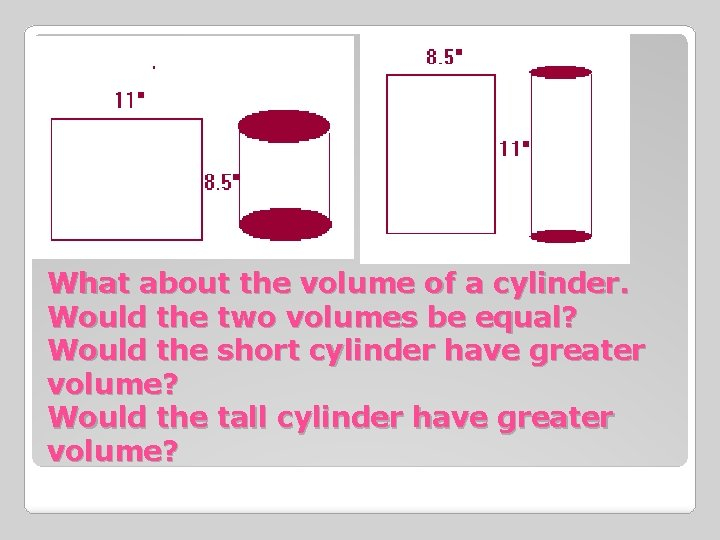What about the volume of a cylinder. Would the two volumes be equal? Would the short cylinder have greater volume? Would the tall cylinder have greater volume?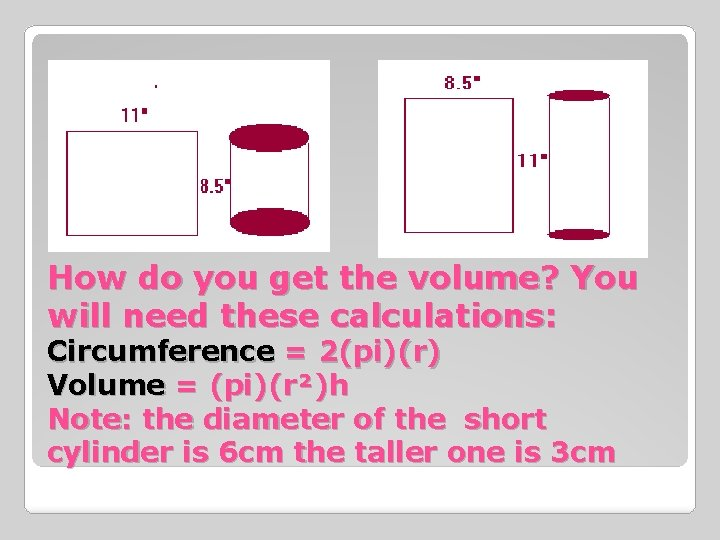How do you get the volume? You will need these calculations: Circumference = 2(pi)(r) Volume = (pi)(r²)h Note: the diameter of the short cylinder is 6 cm the taller one is 3 cm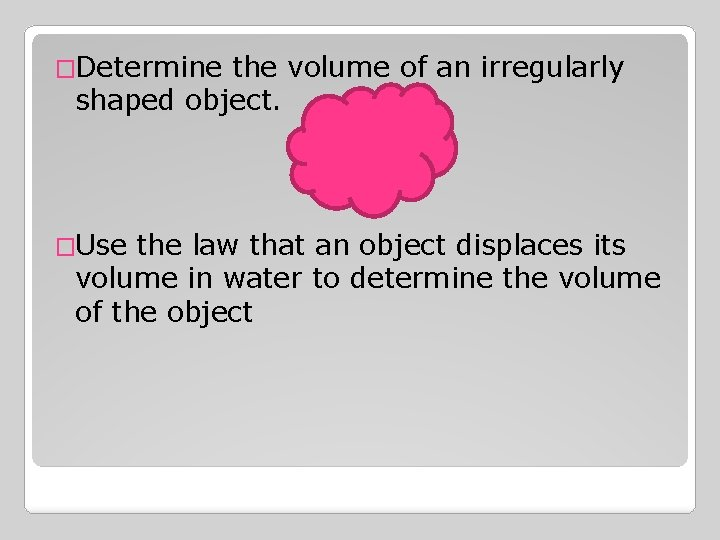�Determine the volume of an irregularly shaped object. �Use the law that an object displaces its volume in water to determine the volume of the object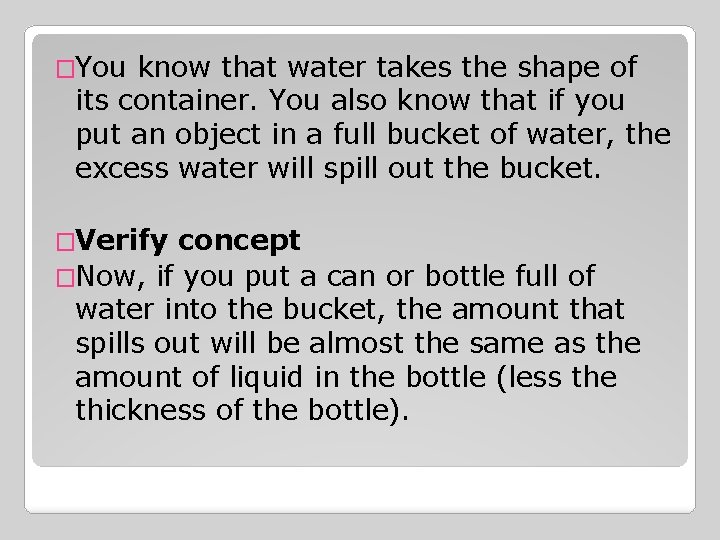�You know that water takes the shape of its container. You also know that if you put an object in a full bucket of water, the excess water will spill out the bucket. �Verify concept �Now, if you put a can or bottle full of water into the bucket, the amount that spills out will be almost the same as the amount of liquid in the bottle (less the thickness of the bottle).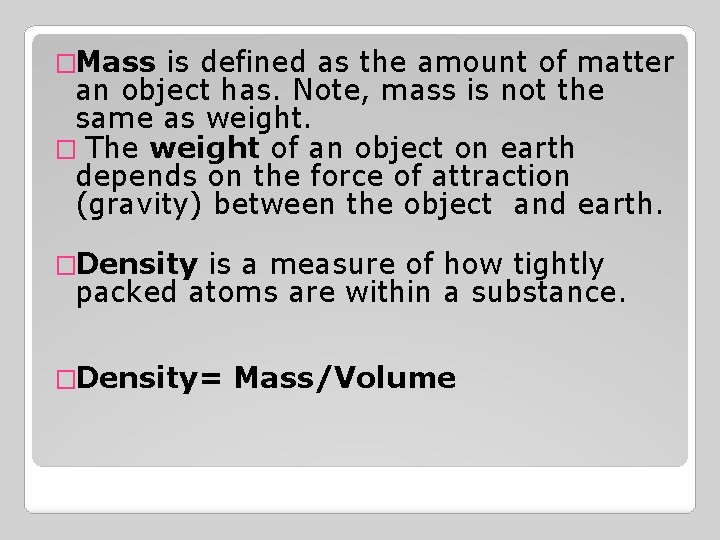�Mass is defined as the amount of matter an object has. Note, mass is not the same as weight. � The weight of an object on earth depends on the force of attraction (gravity) between the object and earth. �Density is a measure of how tightly packed atoms are within a substance. �Density= Mass/Volume�Take a look at the two boxes below. Each box has the same volume. If each ball has the same mass, which box would weigh more? Why? �.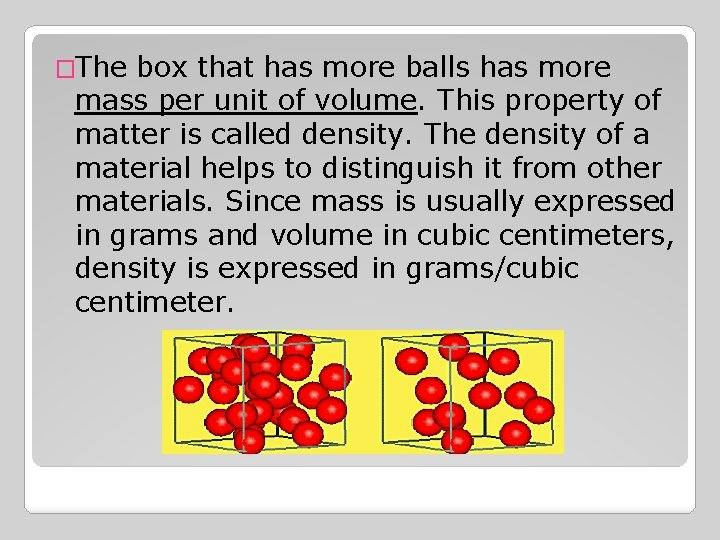�The box that has more balls has more mass per unit of volume. This property of matter is called density. The density of a material helps to distinguish it from other materials. Since mass is usually expressed in grams and volume in cubic centimeters, density is expressed in grams/cubic centimeter.�Calculate the Density( units will be g/cm³) �Block I �Mass = 79. 4 grams �Volume=29. 8 cubic cm. �Block II: �Mass= 25. 4 grams �Volume=29. 8 cubic cm.